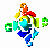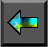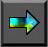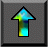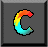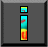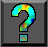Next: Chapter Notes Up: 11 Hypercube Algorithms Previous: 11.5 Summary

# Exercises

1. Execute the hypercube summation algorithm by hand for N=8, and satisfy yourself that you obtain the correct answer.

2. Use Equations 11.1 and 11.2 to identify problem size, processor count, and machine parameter regimes in which each of the two vector reduction algorithms of Section 11.2 will be more efficient.

3. Implement the hybrid vector reduction algorithm described in Section 11.2. Use empirical studies to determine the vector length at which the switch from recursive halving to exchange algorithm should occur. Compare the performance of this algorithm with pure recursive halving and exchange algorithms.

4.   A variant of the parallel mergesort algorithm performs just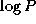compare-exchange operations and then switches to a parallel bubblesort . In the bubblesort phase, tasks are   connected in a logical ring and each task performs compare-exchange operations with its neighbors until a global reduction shows that no exchanges occurred. Design an implementation of this algorithm, using hypercube and ring structures as building blocks.

5. Implement the modified parallel mergesort of Exercise 4. Compare its performance with regular parallel mergesort for different input sequences and for a variety of P and N .

6.   Extend Equations 11.3 and 11.4 to account for bandwidth limitations in a one-dimensional mesh.

7. Modify the performance models developed for the convolution algorithm in Section 4.4 to reflect the use of the hypercube-based transpose. Can the resulting algorithms ever provide superior performance?

8. Use the performance models given in Section 11.2 for the simple and recursive halving vector reduction algorithms to determine situations in which each algorithm would give superior performance.

9. Design and implement a variant of the vector sum algorithm that does not require the number of tasks to be an integer power of 2.

10.   Develop a CC++ , Fortran M, or MPI implementation of a ``hypercube template.'' Use this template to implement simple reduction, vector reduction, and broadcast algorithms. Discuss the techniques that you used to facilitate reuse of the template.

11. Implement a ``torus template'' and use this together with the template developed in Exercise 10 to implement the finite difference computation of Section 4.2.2.

12.   Develop a performance model for a 2-D matrix multiplication algorithm that uses the vector broadcast algorithm of Section 11.2 in place of the tree-based broadcast assumed in Section 4.6.1. Discuss the advantages and disadvantages of this algorithm.

13. Implement both the modified matrix multiplication algorithm of Exercise 12 and the original algorithm of Section 4.6.1, and compare their performance.Next: Chapter Notes Up: 11 Hypercube Algorithms Previous: 11.5 Summary